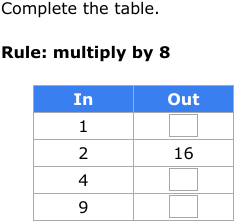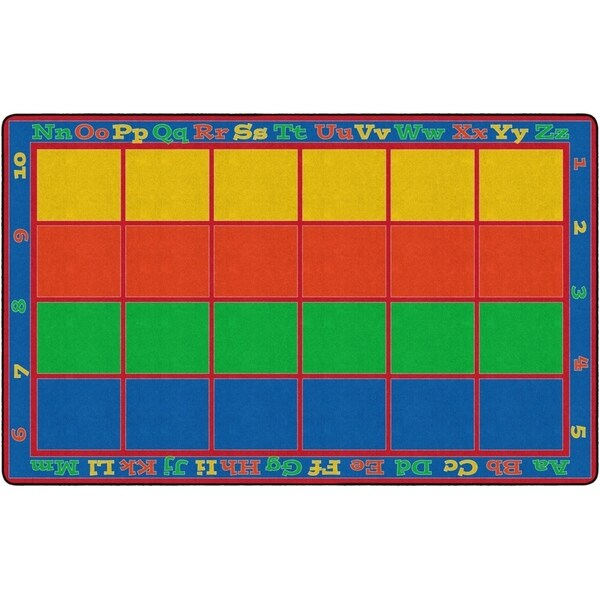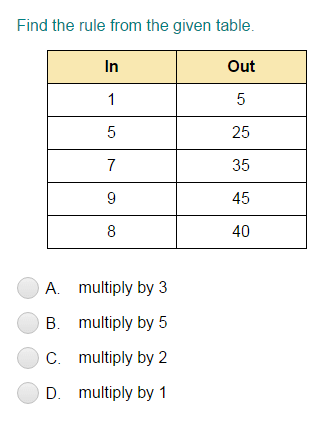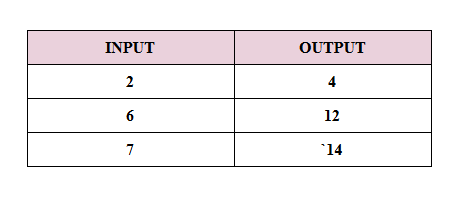Patterns
Multiplication
Division
Story Problems
Fact Family and Fast Facts
1008, 32, 72

100

Which multiplication number sentence represents this array?3 x 5 or 5 x 3

100

Which number sentence best represents

the groups of dots?a. 4 x 20

b. 20 ÷ 5

c. 5 + 4

d. 20 - 4

b. 20 ÷ 5

100

Evan bought packages of 24 cups and put 3 pieces of candy in each cup. How many packages did he buy?

Is this Multiplication or Division?

Division

100

Write a fact family for 6,2,12

6x2=12

2x6=12

12 divided by 6=2

12 divided by 2=6

200If this pattern continued, what would be the 11th output on the chart?

88

200

This carpet shows an area model. Which fact represents the area model shown on the carpet? (HINT: please include the product as well as the fact)4 x 6 = 24 or 6 x 4 = 24

200

Which number sentence is best represented

by this group of stars?a. 12 ÷ 3

b. 3 x 12

c. 3 + 4

d. 12 - 3

a. 12 ÷ 3

200

Jessie and Megan sold 11 cups for \$2.00 a cup. How much money did they make?

Is this Multiplication or Division?

Multiplication

200

Which FOUR facts match the picture below?1. 3 x 5

2. 3 + 3 + 3 + 3 + 3

3. 5 + 5 + 5

4. 5 x 3

5. 5 + 10

6. 15 ÷ 3

3 x 5

5 + 5 + 5

5 x 3

15 ÷ 3

300Multiply by 5

300

Which number sentence is best represented by this number line?2 x 5 = 10

300

Which number sentence is best represented by this number line?15 ÷ 5 = 3

300

Jessie worked for 12 days and worked a total of 144 hours. How many hours did she work each day?

Is this Multiplication or Division?

Division

300

8 x 0 =

0

400

Find the Rule!Multiply by 3

400

Which number sentence is best represented by this number line?3 x 4 = 12

400

Which number sentence is best represented by this number line?24 ÷ 3 = 8

400

There were 72 baked goods for a bake sale. There were 8 customers who all bought the same amount of baked goods. How many baked goods did each customer buy?

Is this Multiplication or Division?

Division

400

What is a division sentence related to 8 x 9 = 72

72 divided by 8 = 9

72 divided by 9 = 8

500

Find the Rule!Multiply by 2
500

12 x F = 12

What is the value of F?

1

500

18 ÷ 1 = N

What is the value of N?

18

500

Mrs. Patience baked 8 cupcakes and each cupcake was 50 calories. How many calories are in the whole batch?

Is this Multiplication or Division?

Multiplication

500

Which basic fact can be used to solve this problem?

5 x 7 = 35

1. 35 - 7 = 28

2. 7 x 35 = 245

1. 35 ÷ 5 = 7

2. 7 + 5 = 12

35 ÷ 5 = 7

Click to zoom